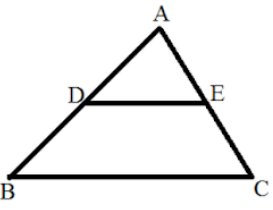Courses
Courses for Kids
Free study material
Offline Centres
MoreLast updated date: 08th Dec 2023
Total views: 384.6k
Views today: 9.84k

# Using theorem 6.1, prove that a line drawn through the midpoint of one side of the triangle parallel to the other side bisects the third side. (Recall that you have proved it in class IX).Theorem 6.1: If a line is drawn parallel to one side of a triangle to intersect the other two sides in distinct points, the other two sides are divided in the same ratio.Verified
In $\vartriangle ABC$, we have given that, D is midpoint of AB and DE is parallel to BC. $\therefore AD = DB$.
We need to prove that AE=EC. Since, $DE\parallel BC$. By basic proportionality theorem, $\dfrac{{AD}}{{DB}} = \dfrac{{AE}}{{EC}}$.
Also, AD=DB so $\dfrac{{AE}}{{EC}} = 1 \Rightarrow AE = EC$. Hence proved.